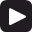# Triangle wave

A triangle wave is a periodic function that increases linearly from a minimum to a maximum and then drops linearly, at the same rate, back to the minimum.

In discrete time, one intuitive way to define the wave is to simply define its pieces. For example, if N is the period of the function, then the function

$$x(k)=\begin{cases}-1+ \frac{k\,mod\,N}{\frac{N}{4}}, k\,mod\,N \le \frac{N}{2} ,\\ 3- \frac{k\,mod\,N}{\frac{N}{4}}, k\,mod\,N \gt \frac{N}{2} \end{cases}$$

is a triangle wave. It increases from -1 to 1 over N/2 samples and then decreases to -1 over the next N/2 samples. Its period is N samples.

There are other ways to define a triangle wave. The following function also produces a triangle wave (subtract N/4 from k to get a triangle wave at the same phase, as the one above).

$$x(k)=\frac{2}{\pi} \, \arcsin(\sin(\frac{2\,\pi\,k}{N}))$$

If, for example, N = 40, then the first triangle wave above will be as follows.The following is a 5 second sample of a triangle wave with the frequency of middle C.

Click Play to hear a triangle wave.## Using triangle waves

Much like square waves and saw waves, triangle waves are rich in harmonics. The following is the discrete Fourier transform of 500 components of the example triangle wave in the graph above.Because of these harmonics – the evenly spaced notches in the graph above – triangle waves are used in synthesizers. To simulate the sound of an instrument, triangle waves (as well as square waves and other) with the desired harmonics can be combined and the result filtered (e.g., through a low pass filter).

## Harmonics of the triangle wave

The peaks in the Fourier transform of a triangle wave with frequency f occur at all odd integer harmonics of f. For example, since the frequency of the triangle wave in the graph above is 50 Hz, the peaks occur at 50 Hz, 150 Hz, 250 Hz, and so on.

In other words, the Fourier series expansion of the triangle wave is an infinite sum of the odd harmonics of the triangle wave frequency (as shown below, each other one is with inverted phase).

## Fourier series expansion of the triangle wave

The Fourier series expansion at sample k of the triangle wave is as follows.

$$\frac{8}{\pi^2} \, \sum_{n=1}^\infty \frac{(-1)^{n+1} \sin(\frac{2\,\pi\,k\,(2n-1)\,f}{f_s})}{n^2}$$

where f is the frequency of the triangle wave and fs is the sampling rate.

The following four graphs show the first term of the series sum, the sum of the first two terms, the sum of the first three terms, and the sum of the first eight terms for f = 33.3 Hz, fs = 16000 Hz and for the first 500 or so samples k on the horizontal axis.The triangle wave exceeds the intended amplitude of 1 at the top and bottom because of the Gibbs phenomenon.

### Filtered HTML

• Freelinking helps you easily create HTML links. Links take the form of [[indicator:target|Title]]. By default (no indicator): Click to view a local node.